Question

# 9. Calculate ΔG0 at 820C for reactions in which (a) ΔH0= 293 kJ; ΔS0 = -695...

9. Calculate ΔG0 at 820C for reactions in which

(a) ΔH0= 293 kJ; ΔS0 = -695 J/K

(b) ΔH0= -1137 kJ; ΔS0= 0.496 kJ/K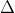G0 =H0 -TS0

We use this formula for both the below questions

T = 820C = (82+273)K = 355K

(a)H0 = 293kJ = 293000J [Since 1kJ = 1000J]G0 =H0 -TS0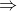G0 = 293000J - (355K x -695J/K)G0 = 293000J + 246725J = 539725J

(b)H0 = -1137kJG0 =H0 -TS0G0 = -1137kJ - (355K x 0.496kJ/K)G0 = -1137kJ - 176.08kJG0 = 1313.08kJ = 131080J

#### Earn Coins

Coins can be redeemed for fabulous gifts.

Similar Homework Help Questions
• ### At what temperatures are the following reactions spontaneous? a) ΔH0 = -35 kJ/mol; ΔS0 = -75...

At what temperatures are the following reactions spontaneous? a) ΔH0 = -35 kJ/mol; ΔS0 = -75 j/Kˑmol b) ΔH0 = -35 kJ/mol; ΔS0 = +75 j/Kˑmol c) ΔH0 = +35 kJ/mol; ΔS0 = -75 j/Kˑmol d) ΔH0 = +35 kJ/mol; ΔS0 = +75 j/Kˑmol

• ### (2 pts) Calculate the equilibrium pressure of CO2 in the system at 3000C. ΔH0 = 88.3...

(2 pts) Calculate the equilibrium pressure of CO2 in the system at 3000C. ΔH0 = 88.3 kJ and ΔS0 = 151.3 J/K. R = 8.314 J/mol K PbCO3(s) ® PbO(s) + CO2(g) ΔH0f(kJ/mol) -699.1 -217.3 -393.5 S0(J/mol K) 131 68.7 213.6

• ### Enter your numbers into 1 decimal places. For the reaction: 4NO(g) + 6H2O(g) ⇋ 4NH3(g) +...Enter your numbers into 1 decimal places. For the reaction: 4NO(g) + 6H2O(g) ⇋ 4NH3(g) + 5O2(g) Determine ΔH° and ΔS° for the reaction at 298 K. ΔH0(kJ) ΔS0(J/K) Assuming that these values are relatively independent of temperature, calculate ΔG° at 100.°C and 2560.°C. ΔG0(kJ)at 100℃   ΔG0(kJ) at 2560℃ 3. Is this reaction spontaneous at all range of temperature? Enter 1 for true or 0 for false.    4. Calculate the K at 298K for the above reaction.  Enter "is" for...

• ### Calculate ΔG0 for the following reactions at 25oC. (a) N2(g) + O2(g) → 2NO(g) ΔG0 =...

Calculate ΔG0 for the following reactions at 25oC. (a) N2(g) + O2(g) → 2NO(g) ΔG0 = kJ/mol (b) H2O(l) → H2O(g) ΔG0 =    kJ/mol (c) 2C2H2(g) + 5O2(g) → 4CO2(g) + 2H2O(l) ΔG0 = kJ/mol

• ### Part A AH = -90 kJ. ASX = - 149 J/K T = 293 K Express...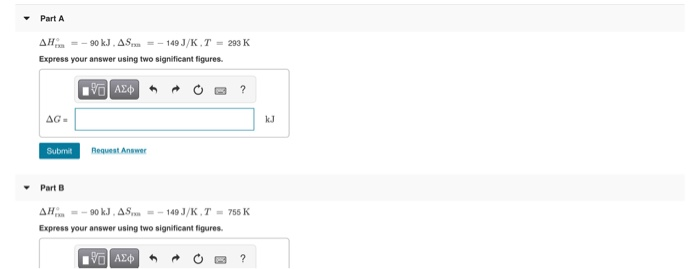Part A AH = -90 kJ. ASX = - 149 J/K T = 293 K Express your answer using two significant figures. AEDRO? AG. Submit Request Answer Part B AH = -90 kJ. AS. = -149 J/K T = 755K Express your answer using two significant figures. 90 ALO R ?

• ### Calculate the ΔH0 for the following reaction: NH4Cl(s) → NH3(g) + HCl(g) Given the following standard...

Calculate the ΔH0 for the following reaction: NH4Cl(s) → NH3(g) + HCl(g) Given the following standard enthalpies of formation ΔHf0 (298 K, 1 atm) NH3(g) -46.2 kJ mol-1 ; HCl(g) -92.3 kJ mol-1 ; NH4Cl(s) -315.0 kJ mol-1

• ### ΔH0 (kJ/mol) C2H2 (g) = 226.7 C6H6 (g) = 82.9 S0(J/mol*K) C2H2 (g) = 200.8 C6H6...

ΔH0 (kJ/mol) C2H2 (g) = 226.7 C6H6 (g) = 82.9 S0(J/mol*K) C2H2 (g) = 200.8 C6H6 (g) = 269.2 Cp C2H2 (g) Cp = 30.7 + 5.28*10-2 T + 1.63*10-5 T2    (J/mol*K) C6H6 (g) Cp = -1.7 + 3.25 *10-2 T - 11.06*10-5 T2 (J/mol*K) Compute ΔH(Temperature Final)reaction and ΔS(Temperature Final)reaction Initial Temperature = 298.15K Final Temperature = 330K

• ### Part AH X = 90 kJ , ASrxn = – 149 J/K , T = 293...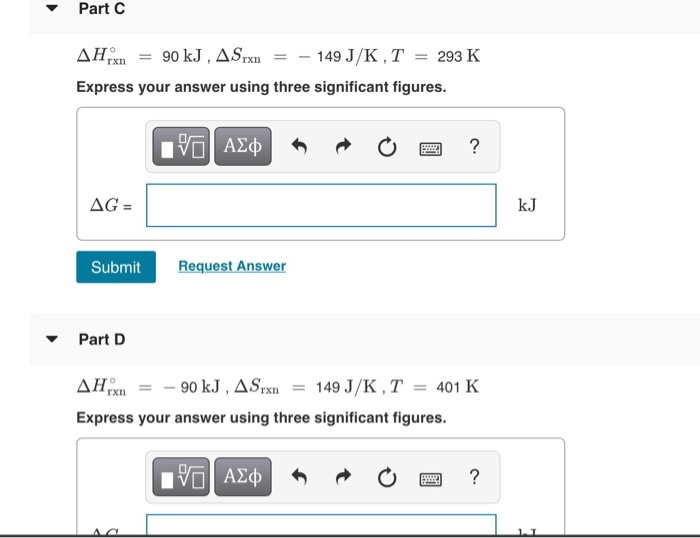Part AH X = 90 kJ , ASrxn = – 149 J/K , T = 293 K Express your answer using three significant figures. 10 AJO O O ? AG = Submit Request Answer Part D AHX = - 90 kJ, A Srxn = 149 J/K, T = 401 K Express your answer using three significant figures. IVO AQ R o o ?

• ### calculate Δh0 ulate AHo (in kJ/mol) for the reaction: B2H6(g)+6 Cl2(g)2 BCls(g)+6 HCl(g) ven the following data: BCI...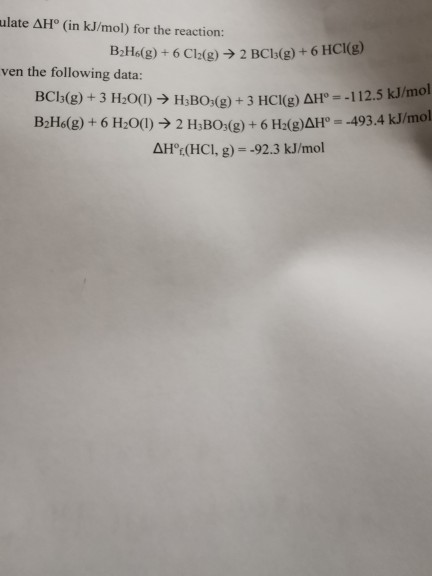calculate Δh0 ulate AHo (in kJ/mol) for the reaction: B2H6(g)+6 Cl2(g)2 BCls(g)+6 HCl(g) ven the following data: BCI5(g)+3 H2O(I) H3BO:(g)+3 HCl(g) AH° = -112.5 kJ/mol B2H&(g) + 6 H2O(1) 2 H3BO:(g) + 6 H2(g)AH°= -493.4 kJ/mol AHOC(HCI, g) -92.3 kJ/mol

• ### Consider the reaction CO(g) + 3H2(9)—CH (9) +H20(9) for which AH° = -206.1 kJ and A...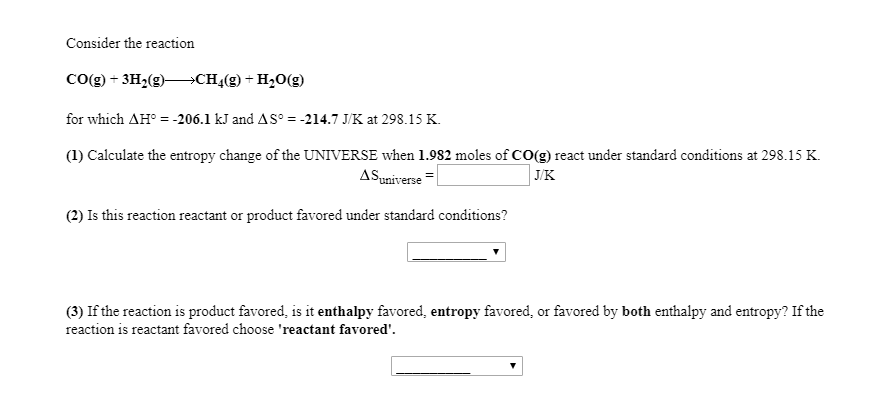Consider the reaction CO(g) + 3H2(9)—CH (9) +H20(9) for which AH° = -206.1 kJ and A Sº = -214.7 J/K at 298.15 K. (1) Calculate the entropy change of the UNIVERSE when 1.982 moles of CO(g) react under standard conditions at 298.15 K. A Suniverse JK (2) Is this reaction reactant or product favored under standard conditions? (3) If the reaction is product favored, is it enthalpy favored, entropy favored, or favored by both enthalpy and entropy? If the reaction...